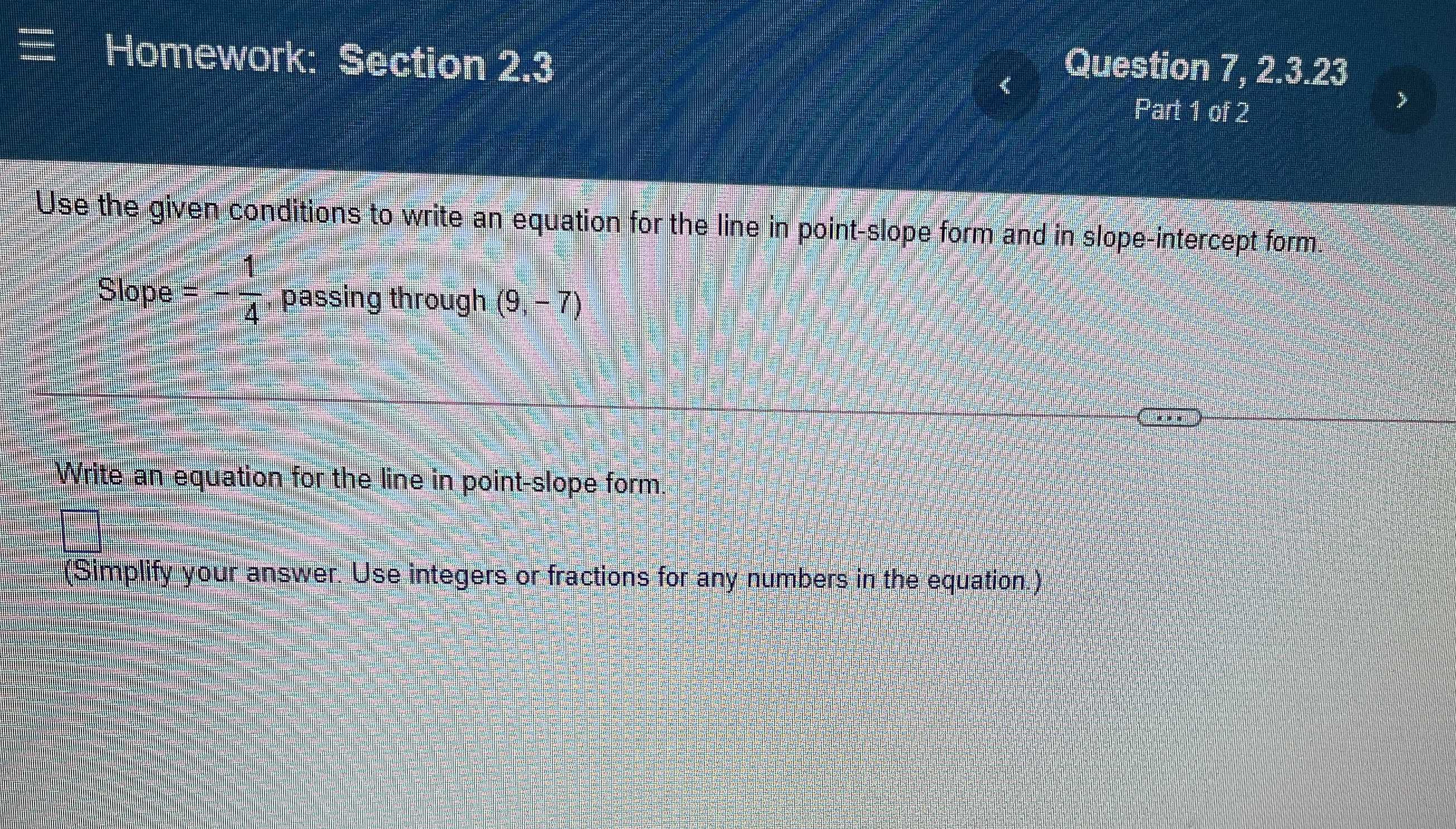### ¿Todavía tienes preguntas de matemáticas?

Pregunte a nuestros tutores expertos
Algebra
PreguntaUse the given conditions to write an equation for the line in point-slope form and in slope-intercept form. Slope $$= - \frac { 1 } { 4 } ,$$ passing through $$( 9 , - 7 )$$ Write an equation for the line in point-slope form. (Simplify your answer. Use integers or fractions for any numbers in the equation.)

y=$$- \frac{1}{4}$$x+b
-7=-$$\frac{1}{4}$$×9+b
b=-$$\frac{19}{4}$$
y=$$- \frac{1}{4}$$x-$$\frac{19}{4}$$